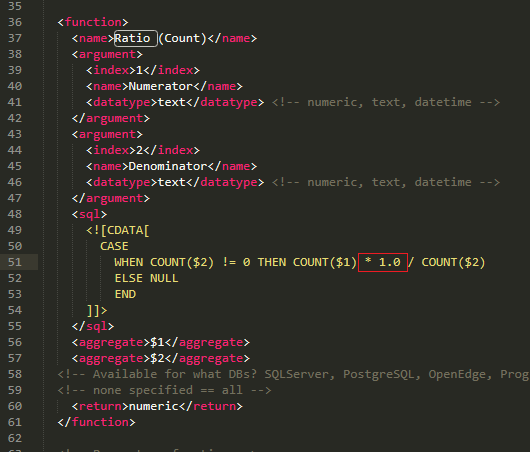# Ratio (Count) pre-built calculated field produces only integer results

Stephen Johnson shared this idea ago
Idea Logged

When I ran into errors with the "Ratio" pre-built function, I tried to use a work around that uses the "Ratio (Count)" pre-built function. This function did not exhibit the same errors as the ratio function, but I discovered it's own limitations. Here is my setup for the pre-built function:And here is the SQL code that gets generated:The problem is that the COUNT() function in SQL always returns an integer. When you do division in SQL between two integers, the result is always rounded to an integer. Very rarely (if ever) would you want to calculate a ratio and have it round to the nearest integer. If I were writing this SQL myself, I would resolve the problem by multiplying either the numerator or denominator by 1.0 to convert that operand to a decimal/float and have my result then be a decimal:The Yellowfin programmers who wrote the Ratio (Count) function apparently didn't think of this, so when I use this function I can only get 1's and 0's and no percentage in between:1

Hi Stephen,

Thanks for reaching out. Whether these values are treated as integers or floats is actually dependent on your driver/RDBMS, but regardless, you can actually edit the underlying equation.

If you head into your <YellowfinInstall>/appserver/webapps/ROOT/WEB-INF folder, you'll see the 'custom-functions.xml' file.

If you go to edit that file you'll see 'Ratio (Count)' as the second function listed there, and in your case, you can simply add "* 1.0", as you mentioned, like so:And then this should work as desired.

Please let me know how this goes and whether you have any further questions.

Regards,

Mike1

What is the use case for rounding the ratio? Am I mistaken in thinking that the use case where you do not want your ratio to be rounded is far, far more common? If not, wouldn't it be better to include this change out of the box for everyone?1

Hi Stephen,

There certainly isn't a good reason I can think of, haha. I too can't see why someone would want a Count, which will almost always be a decimal value, float to be cast as an integer. As of right now the statement as is works the way you'd almost definitely desire it to in MySQL, but does not in MS SQL Server. If the default statement cast the value as a float by adding the "*1.0" all that would mean is that it would work in every RDBMS rather than in some cases, so I think this would make for a valid enhancement request, which I've gone ahead and submitted. I've also changed this to an Idea ticket. Any potential updates regarding this matter will be posted here.

Regards,

Mike